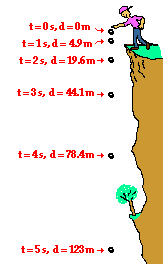1-D Kinematics - Lesson 5 - Free Fall and the Acceleration of Gravity

# How Fast? and How Far?

Free-falling objects are in a state of acceleration. Specifically, they are accelerating at a rate of 9.8 m/s/s. This is to say that the velocity of a free-falling object is changing by 9.8 m/s every second. If dropped from a position of rest, the object will be traveling 9.8 m/s (approximately 10 m/s) at the end of the first second, 19.6 m/s (approximately 20 m/s) at the end of the second second, 29.4 m/s (approximately 30 m/s) at the end of the third second, etc. Thus, the velocity of a free-falling object that has been dropped from a position of rest is dependent upon the time that it has fallen. The formula for determining the velocity of a falling object after a time of t seconds is

vf = g * t

(dropped from rest)

where g is the acceleration of gravity. The value for g on Earth is 9.8 m/s/s. The above equation can be used to calculate the velocity of the object after any given amount of time when dropped from rest. Example calculations for the velocity of a free-falling object after six and eight seconds are shown below.

Example Calculations:

At t = 6 s
vf = (9.8 m/s2) * (6 s) = 58.8 m/s

At t = 8 s
vf = (9.8 m/s2) * (8 s) = 78.4 m/s

The distance that a free-falling object has fallen from a position of rest is also dependent upon the time of fall. This distance can be computed by use of a formula; the distance fallen after a time of t seconds is given by the formula.

d = 0.5 * g * t2

(dropped from rest)

where g is the acceleration of gravity (9.8 m/s/s on Earth). Example calculations for the distance fallen by a free-falling object after one and two seconds are shown below.

Example Calculations:

At t = 1 s
d = (0.5) * (9.8 m/s2) * (1 s)2 = 4.9 m

At t = 2 s
d = (0.5) * (9.8 m/s2) * (2 s)2 = 19.6 m

At t = 5 s
d = (0.5) * (9.8 m/s2) * (5 s)2 = 123 m
(rounded from 122.5 m)

The diagram below (not drawn to scale) shows the results of several distance calculations for a free-falling object dropped from a position of rest.Next Section:
Jump To Next Lesson:

Follow Us